Injection rate profiles, and the discharge and area-contraction coefficients were measured by injecting onto a force transducer and simultaneously collecting and weighing injected fuel (Naber, 1996 and Siebers, 1999). The experimental method and explanation of the orifice coefficients is discussed below.

As the development of a spray is dependent on both the mass and momentum flow rates from an orifice, two orifice coefficients are needed to characterize these flow rates. The discharge coefficient is the product: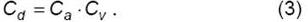where Cv is the velocity coefficient. Using Cv and Ca, the mass flow rate (mf ) and momentum flow rate (Mf ) from an orifice are given by the following: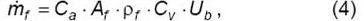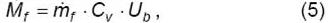where Ub is given by Bernoulli’s equation: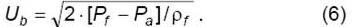The term ρf is the fuel density, Af is the orifice exit area, and Pf and Pa are the fuel and ambient gas pressures, respectively. The velocity Ub is the maximum potential fluid velocity at the orifice exit, while the product Cv·Ub is the average velocity at the orifice exit over the area Ca·Af.

The orifice area-contraction coefficients were determined from the spray momentum measured with a force transducer and the following relationship derived from Eqs. (3-6):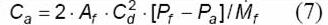The spray momentum was measured with a piezoelectric pressure transducer calibrated to measure the force (i.e., the momentum) induced by a spray impinging on the transducer diaphragm. The transducer was placed approximately 3 mm in front of the orifice. This distance was close enough to the orifice that the entire spray impinged on the central region of the transducer diaphragm, but far enough away that the flow through the orifice was not restricted.

Figure 2.4.1 (0.100 mm data 0.180 mm data) shows injection rates for two orifices with an injection pressure above ambient of 138 MPa and using diesel fuel at room temperature (300 K). The injection period is much longer for the small orifice to inject approximately the same total mass. Injection rates are shown for a single injection, as well as the average of 60 injections. The injection rate profiles show that the opening and closing of the injector occurs in less than 0.1 ms. (The spike at the beginning of injection may be an artifact of mass accumulation at the head of the spray, as the force transducer was a finite distance away from the orifice.) The closing time is shorter for a single injection compared to the mean because of small injection-to-injection variability. Injection is nearly steady during the time of injection, creating a square-wave injection rate shape. The square-wave shape was also found for the off-axis injector tips (Naber, 1996). As opening and closing times are small, the average momentum (Mf) during the steady-flow period was used to determine the orifice coefficients (e.g. Eq.7).